Pre-Calculus

An astronomical unit is the average distance from the earth to the sun. (The earth's orbit is slightly elliptical, so it isn't always the same distance.) The abbreviation is AU.

Saturn is approximately 9.6 AU from the Sun. On the linear scale below, where will Saturn be?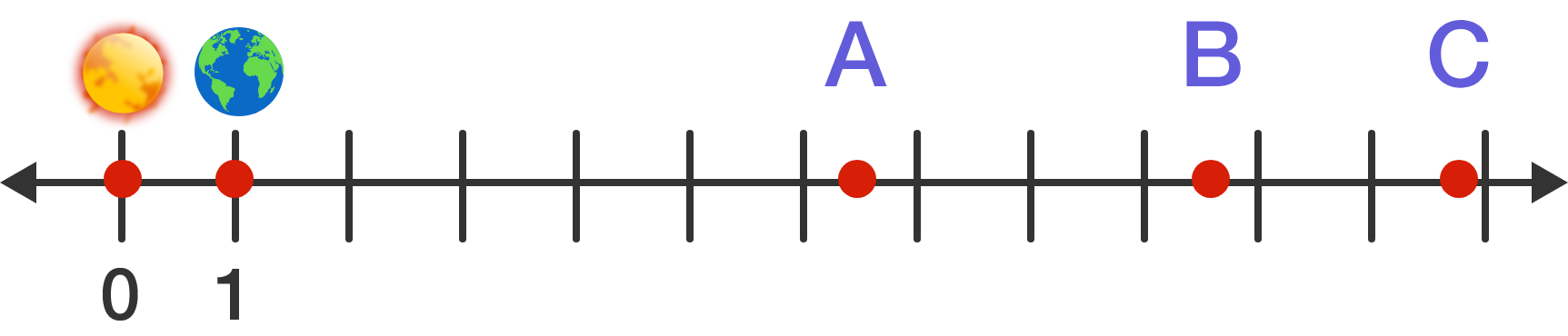Logarithmic Scales in Space

Let's add some more objects to our scale, including the edge of the universe.

 Object Distance from Sun Earth 1 AU Jupiter 5.2 AU Saturn 9.6 AU Heliopause (edge of the solar system) 120 AU Nearest black hole 200,000,000 AU Edge of observable universe 900,000,000,000,000 AU

Which depiction accurately places all the objects on a linear scale?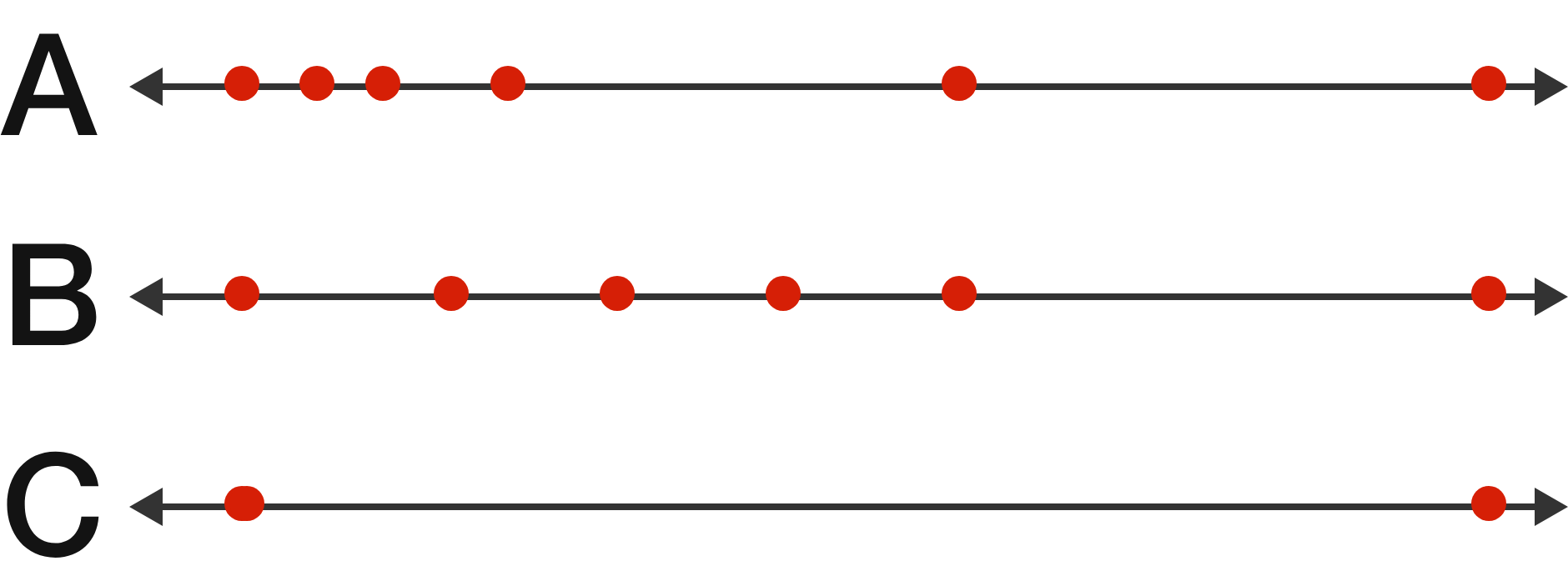Logarithmic Scales in Space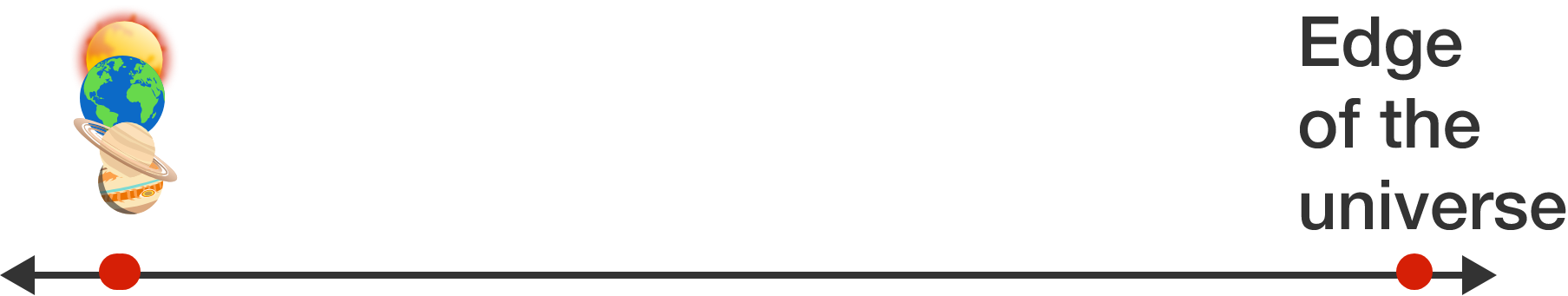Given the entire Solar System essentially collapses on the same point when trying to plot it on a linear scale, a more useful chart is a logarithmic scale.

With a linear scale, moving forward a step adds to the distance a set amount.

With a logarithmic scale, moving forward a step multiplies the distance by a set amount. For the diagram below, each step forward represents a 10-fold multiplication of the distance.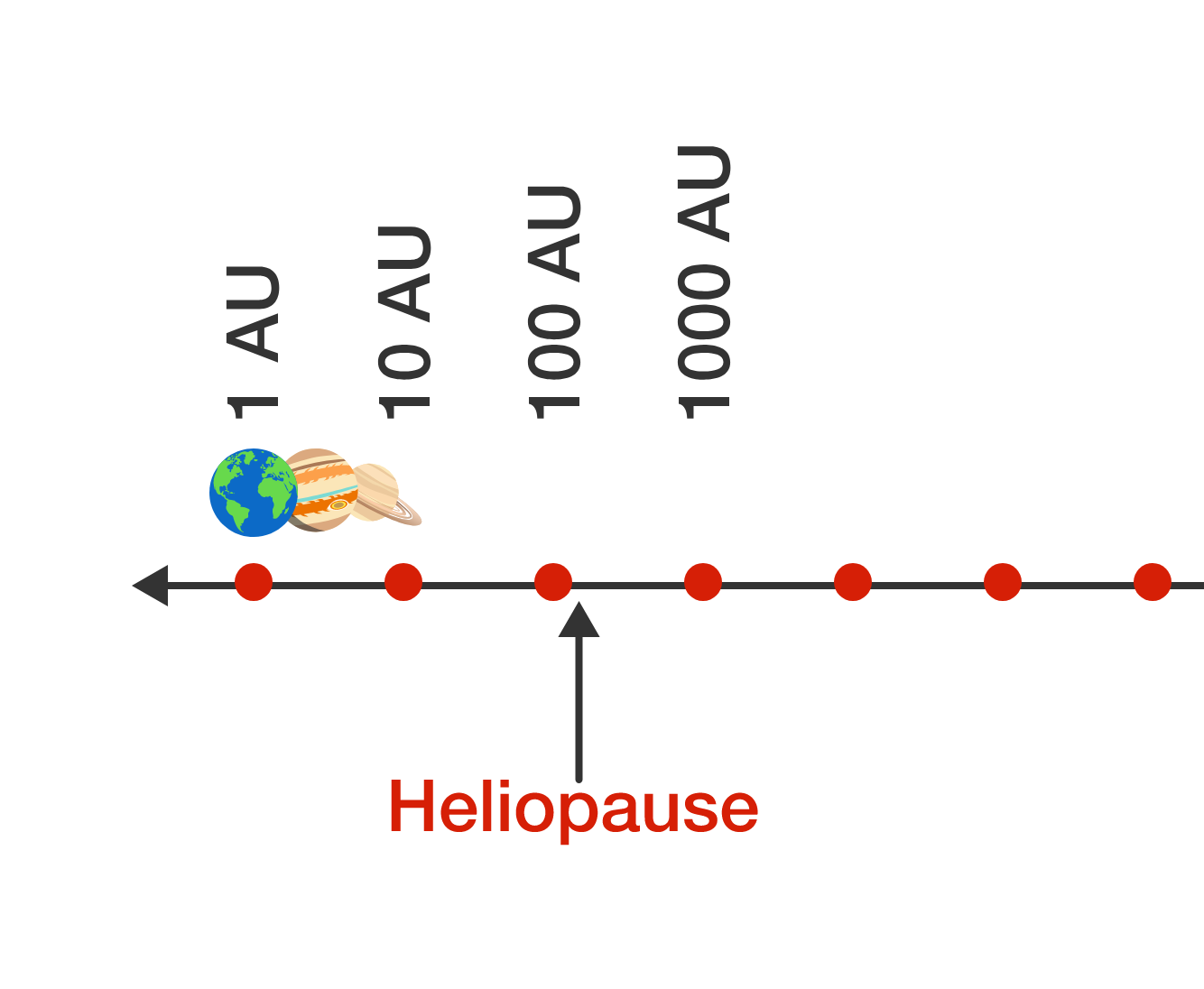The chart above has 7 steps visible. If it continues that pattern as shown, how many steps will the chart need to accurately include 900,000,000,000,000 (900 trillion, or $9 \times 10^{14})$ AU?

Logarithmic Scales in Space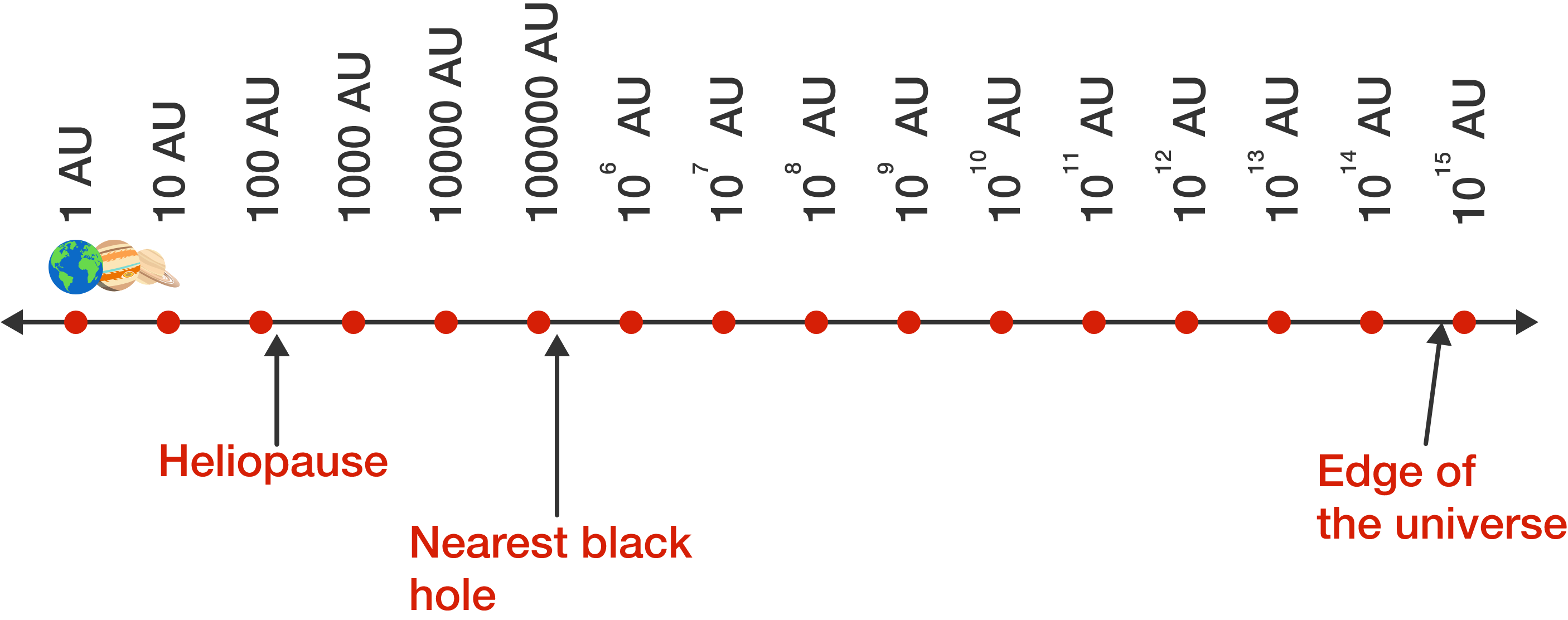If we take the logarithm at a particular point on the chart (past 1 AU), we get how many steps it is along the scale. For example, the logarithm (base 10) of $10^4$ is 4, and that number is 4 steps along the scale.

If you start at some point at distance $D$ from the sun and travel 2 steps to the right, followed by 3 steps, how far are you now from the sun?

Logarithmic Scales in Space

Here's a hint why it's useful to think in terms of logarithmic scales.$\log_{10}(10^3) = 3 \quad \log_{10}(10^2) = 2 \quad \log_{10}(10^3 \times 10^2) = 5$

What expression is equivalent to $\log_{10}(ab) ?$

Logarithmic Scales in Space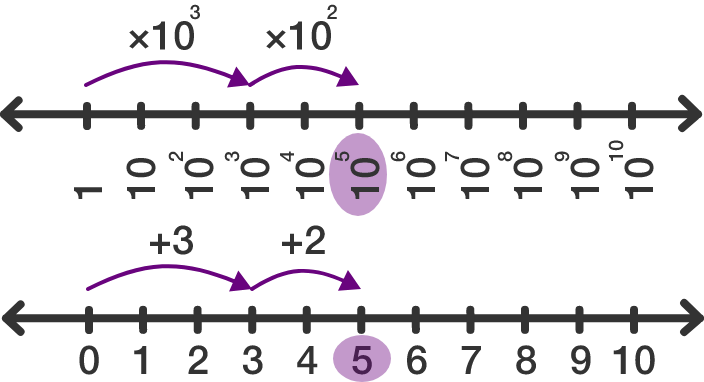Just like we can think of regular arithmetic as shifting around a number line, we can think of logarithm arithmetic as being done on a logarithmic scale. We can derive all the rules for logarithms starting from this idea.

Pre-Calculus can be done naturally and intuitively: get started and find out how!

×